# Kaslo Bull Trout Productivity 2019

The suggested citation for this analytic appendix is:

Thorley, J.L., Amies-Galonski, E. and Dalgarno, S.I.J. (2019) Kaslo Bull Trout Productivity 2019. A Poisson Consulting Analysis Appendix. URL: https://www.poissonconsulting.ca/f/168922378.

## Background

The Kaslo River and Keen Creek, which is a tributary of the Kaslo River, are important Bull Trout spawning and rearing tributaries on Kootenay Lake. From 2012 to 2019, field crews have night-snorkeled both systems in the fall and recorded all juvenile Bull Trout less than 350 mm in length. Snorkel and electrofishing marking crews have also captured and tagged juvenile Bull Trout for the snorkel crews to resight. Redd counts have been conducted in both systems since 2006.

The primary goal of the current analyses is to answer the following questions:

What is the observer efficiency when night-snorkeling for juvenile Bull Trout in the Kaslo River and Keen Creek?

What are the densities of age-1 Bull Trout in the Kaslo River and Keen Creek?

What is the relationship between the number of redds and the resultant densities of age-1 Bull Trout two years later?

### Data Preparation

The data were cleaned, tidied and databased using R version 3.6.1 (R Core Team 2015).

### Statistical Analysis

Model parameters were estimated using Maximum-Likelihood (ML) and Bayesian methods. The Maximum-Likelihood Estimates (MLE) were obtained using TMB (Kristensen et al. 2016) while the Bayesian estimates were produced using JAGS (Plummer 2015). For additional information on ML and Bayesian estimation the reader is referred to Millar (2011) and McElreath (2016), respectively.

Unless indicated otherwise, the Bayesian analyses used uninformative normal prior distributions (Kery and Schaub 2011, 36). The posterior distributions were estimated from 1500 Markov Chain Monte Carlo (MCMC) samples thinned from the second halves of 3 chains (Kery and Schaub 2011, 38–40). Model convergence was confirmed by ensuring that $$\hat{R} \leq 1.1$$ (Kery and Schaub 2011, 40) and $$\textrm{ESS} \geq 150$$ for each of the monitored parameters (Kery and Schaub 2011, 61). Where $$\hat{R}$$ is the potential scale reduction factor and $$\textrm{ESS}$$ is the effective sample size.

The parameters are summarised in terms of the point estimate, standard deviation (sd), the z-score, lower and upper 95% confidence/credible limits (CLs) and the p-value (Kery and Schaub 2011, 37, 42). For ML models, the point estimate is the MLE, the standard deviation is the standard error, the z-score is $$\mathrm{sd}/\mathrm{MLE}$$ and the 95% CLs are the $$\mathrm{MLE} \pm 1.96 \cdot \mathrm{sd}$$. For Bayesian models, the estimate is the median (50th percentile) of the MCMC samples, the z-score is $$\mathrm{sd}/\mathrm{mean}$$ and the 95% CLs are the 2.5th and 97.5th percentiles. A p-value of 0.05 indicates that the lower or upper 95% CL is 0.

Model averaging and selection was implemented using an information theoretic approach (Burnham and Anderson 2002). ML models were compared using the marginal Akaike’s Information Criterion corrected for small sample size (AICc, Burnham and Anderson 2002). The support for fixed terms in models with identical random (Kery and Schaub 2011, 75) effects was assessed using the Akaike’s weights ($$w_i$$) (Burnham and Anderson 2002). The best supported model was then refitted as its Bayesian equivalent.

Where relevant, model adequacy was confirmed by examination of residual plots for the full model(s).

The results are displayed graphically by plotting the modeled relationships between particular variables and the response(s) with the remaining variables held constant. In general, continuous and discrete fixed variables are held constant at their mean and first level values, respectively, while random variables are held constant at their typical values (expected values of the underlying hyperdistributions) (Kery and Schaub 2011, 77–82). When informative the influence of particular variables is expressed in terms of the effect size (i.e., percent change in the response variable) with 95% confidence/credible intervals (CIs, Bradford, Korman, and Higgins 2005).

The analyses were implemented using R version 3.6.1 (R Core Team 2015) and the mbr family of packages.

### Model Descriptions

#### Length Correction

The annual bias (inaccuracy) and error (imprecision) in observer’s fish length estimates when spotlighting (standing) and snorkeling were quantified from the divergence of their length distribution from the length distribution for JLT and SH (the two most experience snorkelers) in that year. More specifically, the length correction that minimised the Jensen-Shannon divergence (Lin 1991) provided a measure of the inaccuracy while the minimum divergence (the Jensen-Shannon divergence was calculated with log to base 2 which means it lies between 0 and 1) provided a measure of the imprecision.

#### Observer Efficiency

All resighted fish with a tag were allocated to the closest unallocated marked fish (with the same colour tag) by fork length and distance. The marked fish were analysed using a logistic regression model. Key assumptions of the full Maximum Likelihood logistic regression include:

• The observer efficiency varies by the fork length as a second order polynomial.
• The observer efficiency varies by observer.
• The observer efficiency varies between systems.
• The observer efficiency varies by the gradient, sinuosity and river kilometre.

Preliminary analysis indicated that river kilometre received similar support to watershed area.

The final Bayesian logistic regression assumed that:

• The observer efficiency varies by the fork length.

#### Lineal Density

Both systems were broken into 100 m sites by bank. The lineal density at each site was estimated using an over-dispersed Poisson Generalized Linear Mixed Model. Key assumptions of the full Maximum Likelihood GLMM include:

• The lineal density varies between systems and years.
• The lineal density varies randomly by site.
• The lineal density varies by site sinuosity, gradient and river kilometre.
• The observer efficiency for each system is as estimated by the observer efficiency model.

Preliminary analysis indicated that river kilometre was better supported as a predictor of lineal density than watershed area. Preliminary analysis also indicated that autocorrelation (modeled as an AR1 process) was not significant.

The final Bayesian GLMM dropped sinuosity and gradient and took into account the uncertainty in the observer efficiencies as estimated by the observer efficiency model.

#### Stock-Recruitment

The stock-recruitment relationship was estimated using a Bayesian Beverton-Holt stock-recruitment curve. Key assumptions of the final BH SR model include:

• The strength of density-dependence varies between systems.

### Model Templates

#### Observer Efficiency

##### Maximum Likelihood
.#include <TMB.hpp>

template<class Type>
Type objective_function<Type>::operator() () {
DATA_VECTOR(Observed);
DATA_FACTOR(Observer);
DATA_FACTOR(System);
DATA_VECTOR(Tagged);
DATA_VECTOR(Length);
DATA_VECTOR(Gradient);
DATA_VECTOR(Sinuosity);
DATA_VECTOR(Rkm);

PARAMETER(bSystem);
PARAMETER(bLength);
PARAMETER(bLength2);
PARAMETER(bGradient);
PARAMETER(bSinuosity);
PARAMETER(bRkm);

PARAMETER_VECTOR(bObserver);

vector<Type> eObserved = Observed;

int n = Observed.size();

Type nll = 0.0;

for(int i = 0; i < n; i++){
eObserved(i) = invlogit(bObserver(Observer(i)) + bSystem * System(i) + bLength * Length(i) + bLength2 * pow(Length(i), 2) + bGradient * Gradient(i) + bSinuosity * Sinuosity(i) + bRkm * Rkm(i));
nll -= dbinom(Observed(i), Tagged(i), eObserved(i), true);
return nll;
..

Block 1. Full model.

##### Bayesian
.model{
bIntercept ~ dnorm(0, 5^-2)
bLength ~ dnorm(0, 5^-2)

for(i in 1:length(Observed)){
logit(eObserved[i]) <-  bIntercept + bLength * Length[i]
Observed[i] ~ dbern(eObserved[i])
}
..

Block 2. Final model.

#### Lineal Density

##### Maximum Likelihood
.#include <TMB.hpp>

template<class Type>
Type objective_function<Type>::operator() () {
DATA_VECTOR(Count);
DATA_VECTOR(Length);
DATA_VECTOR(Coverage);
DATA_VECTOR(Efficiency);
DATA_VECTOR(Dispersion);

DATA_VECTOR(Gradient);
DATA_VECTOR(Sinuosity);
DATA_VECTOR(Rkm);

DATA_FACTOR(System);

DATA_FACTOR(Site);
DATA_INTEGER(nSite);
DATA_FACTOR(Year);

PARAMETER_MATRIX(bSystemYear);
PARAMETER_VECTOR(bSite);

PARAMETER(bGradient);
PARAMETER(bSinuosity);
PARAMETER(bRkm);

PARAMETER_VECTOR(bDispersion);
DATA_INTEGER(nDispersion);
PARAMETER(log_sDispersion);
PARAMETER(log_sSite);

Type sSite = exp(log_sSite);
Type sDispersion = exp(log_sDispersion);

ADREPORT(sSite);
ADREPORT(sDispersion);

vector<Type> eDensity = Count;
vector<Type> eCount = Count;
vector<Type> eNorm = pnorm(bDispersion, Type(0), Type(1));
vector<Type> eDispersion = qgamma(eNorm, pow(sDispersion, -2), pow(sDispersion, 2));

Type nll = 0.0;

for(int i = 0; i < nSite; i++){
nll -= dnorm(bSite(i), Type(0), sSite, true);

for(int i = 0; i < nDispersion; i++){
nll -= dnorm(bDispersion(i), Type(0), Type(1), true);

eDensity(i) = exp(bSystemYear(System(i), Year(i)) + bGradient * Gradient(i) + bSinuosity * Sinuosity(i) +  bRkm * Rkm(i) + bSite(Site(i)));
eCount(i) = eDensity(i) * Length(i) * Coverage(i);

nll -= dpois(Count(i), eCount(i) * Efficiency(i) * eDispersion(i), true);

return nll;
..

Block 3. The full model.

##### Bayesian
.model{

bEfficiency ~ dnorm(Efficiency, EfficiencySD^-2) T(0, )

for(i in 1:nSystem) {
for(j in 1:nYear) {
bSystemYear[i,j] ~ dnorm(0, 5^-2)
}
}

log_sSite ~ dnorm(0, 5^-2)
log(sSite) <- log_sSite
for(i in 1:nSite) {
bSite[i] ~ dnorm(0, sSite^-2)
}

bRkm ~ dnorm(0, 5^-2)

log_sDispersion ~ dnorm(0, 5^-2)
log(sDispersion) <- log_sDispersion

for(i in 1:length(Count)) {
log(eDensity[i]) <- bSystemYear[System[i],Year[i]] + bRkm * Rkm[i] + bSite[Site[i]]
eDispersion[i] ~ dgamma(sDispersion^-2, sDispersion^-2)
Count[i] ~ dpois(eDensity[i] * Length[i] * Coverage[i] * bEfficiency * eDispersion[i])
}
..

Block 4. The final model.

#### Stock-Recruiment

.model {
log_bAlpha ~ dnorm(5, 5^-2)
log(bAlpha) <- log_bAlpha

for(i in 1:nSystem) {
log_bBeta[i] ~ dnorm(0, 5^-2)
log(bBeta[i]) <- log_bBeta[i]
}

log_sRecruits ~ dnorm(0, 5^-2)
log(sRecruits) <- log_sRecruits

for(i in 1:length(Recruits)){
eRecruits[i] <- bAlpha * Stock[i] / (1 + bBeta[System[i]] * Stock[i])
Recruits[i] ~ dlnorm(log(eRecruits[i]), sRecruits^-2)
}
bCarryingCapacity <- bAlpha / bBeta
..

Block 5. Final model.

## Results

### Tables

#### Coverage

Table 1. Total length of river bank counted (including replicates) by system and year.

System Year Length (km)
Kaslo River 2012 10.57 [km]
Kaslo River 2013 13.43 [km]
Kaslo River 2014 11.45 [km]
Kaslo River 2015 7.32 [km]
Kaslo River 2016 11.59 [km]
Kaslo River 2017 9.79 [km]
Kaslo River 2018 8.43 [km]
Kaslo River 2019 7.19 [km]
Keen Creek 2012 1.44 [km]
Keen Creek 2013 0.95 [km]
Keen Creek 2014 0.67 [km]
Keen Creek 2015 0.72 [km]
Keen Creek 2016 0.85 [km]
Keen Creek 2017 1.68 [km]
Keen Creek 2018 3.37 [km]
Keen Creek 2019 1.48 [km]

#### Observer Efficiency

Table 2. Parameter descriptions.

Parameter Description
bGradient The effect of Gradient on bIntercept
bIntercept The intercept for logit(eObserved)
bLength The effect of Length on bIntercept
bLength2 The effect of Length on the effect of Length on bIntercept
bRkm The effect of Rkm on bIntercept
bSinuosity The effect of Sinuosity on bIntercept
eObserved The expected probability of observing an individual
Gradient The standardized site-level gradient
Length The standardized fork length
Observed The number of individuals observed (0 or 1)
Rkm The standardized Rkm
Sinuosity The standardized site-level sinuosity
Tagged The number of tagged individuals (1)
##### Maximum Likelihood

Table 3. Model averaged parameter estimates and Akaike weights.

term estimate sd zscore lower upper pvalue nmodels proportion ICWt
bGradient 0.0918623 0.1509254 0.6086601 -0.2039461 0.3876706 0.5427498 96 0.5000000 0.437
bIntercept -1.0808780 0.6371123 -1.6965266 -2.3295953 0.1678392 0.0897862 96 0.5000000 0.755
bLength 0.6677614 0.2038890 3.2751216 0.2681462 1.0673766 0.0010562 96 0.6666667 0.993
bLength2 -0.0425534 0.1231498 -0.3455416 -0.2839225 0.1988157 0.7296872 96 0.3333333 0.311
bObserver -2.3082666 27.3607587 -0.0843641 -55.9343683 51.3178350 0.9327669 96 0.5000000 0.238
bObserver -0.3257582 0.6112646 -0.5329250 -1.5238149 0.8722984 0.5940855 96 0.5000000 0.238
bObserver -0.3072008 0.5629593 -0.5456891 -1.4105807 0.7961792 0.5852797 96 0.5000000 0.238
bObserver -2.2142360 25.2858171 -0.0875683 -51.7735269 47.3450548 0.9302198 96 0.5000000 0.238
bObserver -0.3711566 0.8717147 -0.4257777 -2.0796860 1.3373728 0.6702699 96 0.5000000 0.238
bRkm -0.0126214 0.1135448 -0.1111581 -0.2351652 0.2099224 0.9114910 96 0.5000000 0.268
bSinuosity -0.0094249 0.0883606 -0.1066638 -0.1826084 0.1637587 0.9150557 96 0.5000000 0.262
bSystem -0.0278483 0.2590061 -0.1075198 -0.5354908 0.4797943 0.9143766 96 0.5000000 0.269
##### Bayesian

Table 4. Final parameter estimates.

term estimate sd zscore lower upper pvalue
bIntercept -1.4809882 0.1929421 -7.676471 -1.8642345 -1.138266 7e-04
bLength 0.6890303 0.2013763 3.464707 0.3319783 1.101899 7e-04

Table 5. Observer Efficiency estimates for a 123 mm Bull Trout.

System Efficiency EfficiencySD EfficiencyLower EfficiencyUpper
Kaslo River 0.1474655 0.0292574 0.0960496 0.207584

Table 6. Final model summary.

n K nchains niters nthin ess rhat converged
220 2 3 500 1 573 1.005 TRUE

#### Lineal Density

Table 7. Parameter descriptions.

Parameter Description
bDispersion The random effect of overdispersion
bGradient The effect of Gradient on bSystemYear
bRkm The effect of Rkm on bSystemYear
bSinuosity The effect of Sinuosity on bSystemYear
bSite The random effect of Site on bSystemYear
bSystemYear The effect of System and Year on log(eDensity)
Count Number of fish counted
Coverage Proportion of site surveyed
Dispersion Factor for random effect of overdispersion
eCount The expected Count
eDensity The expected lineal density
Efficiency The observer efficiency from the observer efficiency model
Gradient Standardized gradient
Length Length of site (m)
log_sDispersion log(sDispersion)
log_sSite log(sSite)
Rkm Standardized Rkm
sDispersion The SD of bDispersion
Sinuosity Standardized sinuosity
Site The site
sSite The SD of bSite
System The system
Year The year
##### Maximum Likelihood

Table 8. Model averaged parameter estimates and Akaike weights.

term estimate sd zscore lower upper pvalue nmodels proportion ICWt
bGradient 0.0311395 0.0454417 0.6852622 -0.0579247 0.1202037 0.4931785 8 0.5 0.482
bRkm 0.4715056 0.0547874 8.6060899 0.3641242 0.5788871 0.0000000 8 0.5 0.999
bSinuosity 0.0363242 0.0456070 0.7964614 -0.0530639 0.1257123 0.4257639 8 0.5 0.545
bSystemYear[1,1] -2.6455437 0.1121992 -23.5789813 -2.8654502 -2.4256373 0.0000000 8 1.0 0.999
bSystemYear[2,1] -1.2172578 0.2447367 -4.9737450 -1.6969329 -0.7375827 0.0000007 8 1.0 0.999
bSystemYear[1,2] -2.8589534 0.1051397 -27.1919385 -3.0650235 -2.6528833 0.0000000 8 1.0 0.999
bSystemYear[2,2] -1.5767460 0.3377544 -4.6683203 -2.2387325 -0.9147594 0.0000030 8 1.0 0.999
bSystemYear[1,3] -2.9864266 0.1161414 -25.7137179 -3.2140595 -2.7587937 0.0000000 8 1.0 0.999
bSystemYear[2,3] -1.1402911 0.3443506 -3.3114251 -1.8152058 -0.4653764 0.0009282 8 1.0 0.999
bSystemYear[1,4] -2.4212448 0.1254062 -19.3072247 -2.6670364 -2.1754533 0.0000000 8 1.0 0.999
bSystemYear[2,4] -0.5882569 0.3099003 -1.8982133 -1.1956504 0.0191365 0.0576680 8 1.0 0.999
bSystemYear[1,5] -2.8513065 0.1148164 -24.8336078 -3.0763426 -2.6262704 0.0000000 8 1.0 0.999
bSystemYear[2,5] -2.7100919 0.5109829 -5.3036836 -3.7116000 -1.7085837 0.0000001 8 1.0 0.999
bSystemYear[1,6] -2.1502622 0.0983227 -21.8694488 -2.3429711 -1.9575533 0.0000000 8 1.0 0.999
bSystemYear[2,6] -1.3561990 0.2486467 -5.4543206 -1.8435377 -0.8688604 0.0000000 8 1.0 0.999
bSystemYear[1,7] -2.5353756 0.1163632 -21.7884632 -2.7634433 -2.3073079 0.0000000 8 1.0 0.999
bSystemYear[2,7] -1.6811798 0.2023087 -8.3099714 -2.0776976 -1.2846620 0.0000000 8 1.0 0.999
bSystemYear[1,8] -2.6358901 0.1298255 -20.3033252 -2.8903434 -2.3814367 0.0000000 8 1.0 0.999
bSystemYear[2,8] -1.7410385 0.2792599 -6.2344738 -2.2883778 -1.1936992 0.0000000 8 1.0 0.999
log_sDispersion -0.6849019 0.1069518 -6.4038346 -0.8945236 -0.4752801 0.0000000 8 1.0 0.999
log_sSite -0.8235911 0.1355269 -6.0769561 -1.0892190 -0.5579633 0.0000000 8 1.0 0.999
##### Bayesian

Table 9. Parameter estimates.

term estimate sd zscore lower upper pvalue
bEfficiency 0.1378714 0.0298430 4.580539 0.0741621 0.1939628 0.0007
bRkm 0.4783569 0.0556769 8.568303 0.3671965 0.5855429 0.0007
bSystemYear[1,1] -2.5719482 0.2662244 -9.535631 -2.9771583 -1.9249779 0.0007
bSystemYear[2,1] -1.0970516 0.3484160 -3.097938 -1.7267405 -0.3472264 0.0080
bSystemYear[1,2] -2.7818108 0.2597451 -10.617058 -3.2019452 -2.1599471 0.0007
bSystemYear[2,2] -1.4362010 0.4259282 -3.330629 -2.2011092 -0.5812363 0.0053
bSystemYear[1,3] -2.9255387 0.2684283 -10.783027 -3.3525650 -2.2922316 0.0007
bSystemYear[2,3] -1.0137786 0.4060204 -2.416645 -1.7259986 -0.1512328 0.0227
bSystemYear[1,4] -2.3400137 0.2682543 -8.619585 -2.7778505 -1.7078437 0.0007
bSystemYear[2,4] -0.4341576 0.4031108 -1.034867 -1.1468397 0.4401022 0.2907
bSystemYear[1,5] -2.7836306 0.2688845 -10.241027 -3.2140260 -2.1472869 0.0007
bSystemYear[2,5] -2.5888360 0.5501223 -4.725784 -3.7170614 -1.5263210 0.0007
bSystemYear[1,6] -2.0849773 0.2552975 -8.040223 -2.4775737 -1.4508127 0.0007
bSystemYear[2,6] -1.2145196 0.3426224 -3.516282 -1.8631924 -0.4959314 0.0027
bSystemYear[1,7] -2.4405574 0.2646835 -9.155318 -2.8815792 -1.8697727 0.0007
bSystemYear[2,7] -1.5533202 0.3127407 -4.912500 -2.0925553 -0.8532112 0.0007
bSystemYear[1,8] -2.5728975 0.2777654 -9.151591 -3.0097806 -1.9068394 0.0007
bSystemYear[2,8] -1.6155660 0.3686062 -4.362258 -2.3086733 -0.8543728 0.0007
log_sDispersion -0.6079784 0.1030649 -5.957853 -0.8318048 -0.4140571 0.0007
log_sSite -0.8346942 0.1538637 -5.510836 -1.1895857 -0.6001209 0.0007

Table 10. Model summary.

n K nchains niters nthin ess rhat converged
982 20 3 500 20 134 1.019 FALSE

#### Stock-Recruiment

Table 11. Parameter descriptions.

Parameter Description
bBeta Density-dependence
eRecruits Expected value of Recruits
Recruits Number of age-1 Bull Trout
Stock Number of Bull Trout redds
bAlpha Recruits per stock at low stock density
sRecruits SD of residual variation in Recruits
##### Bayesian

Table 12. Final parameter estimates.

term estimate sd zscore lower upper pvalue
bAlpha 107.9617072 6.959718e+03 0.1256190 33.5430430 3998.2911962 0.0007
bBeta 0.4966219 3.690286e+01 0.1224021 0.0769660 23.7598897 0.0007
bBeta 0.2378008 2.501060e+01 0.1184190 0.0030618 13.2126752 0.0007
bCarryingCapacity 224.2683120 9.128826e+02 0.2946850 149.2815777 488.7363168 0.0007
bCarryingCapacity 456.6886954 6.821273e+05 0.0336592 244.7990487 11760.8229020 0.0007
log_bAlpha 4.6817766 1.210229e+00 4.1163117 3.5128293 8.2933643 0.0007
log_bBeta -0.6999266 1.388835e+00 -0.3402677 -2.5644317 3.1679938 0.5347
log_bBeta -1.4363280 1.932357e+00 -0.7136175 -5.7887633 2.5811351 0.3373
log_sRecruits -0.8019392 2.074041e-01 -3.7961545 -1.1573710 -0.3456840 0.0007
sRecruits 0.4484585 1.005801e-01 4.6247301 0.3143116 0.7077362 0.0007

Table 13. Final model summary.

n K nchains niters nthin ess rhat converged
16 10 3 500 100 288 1.012 TRUE

### Figures

#### Systems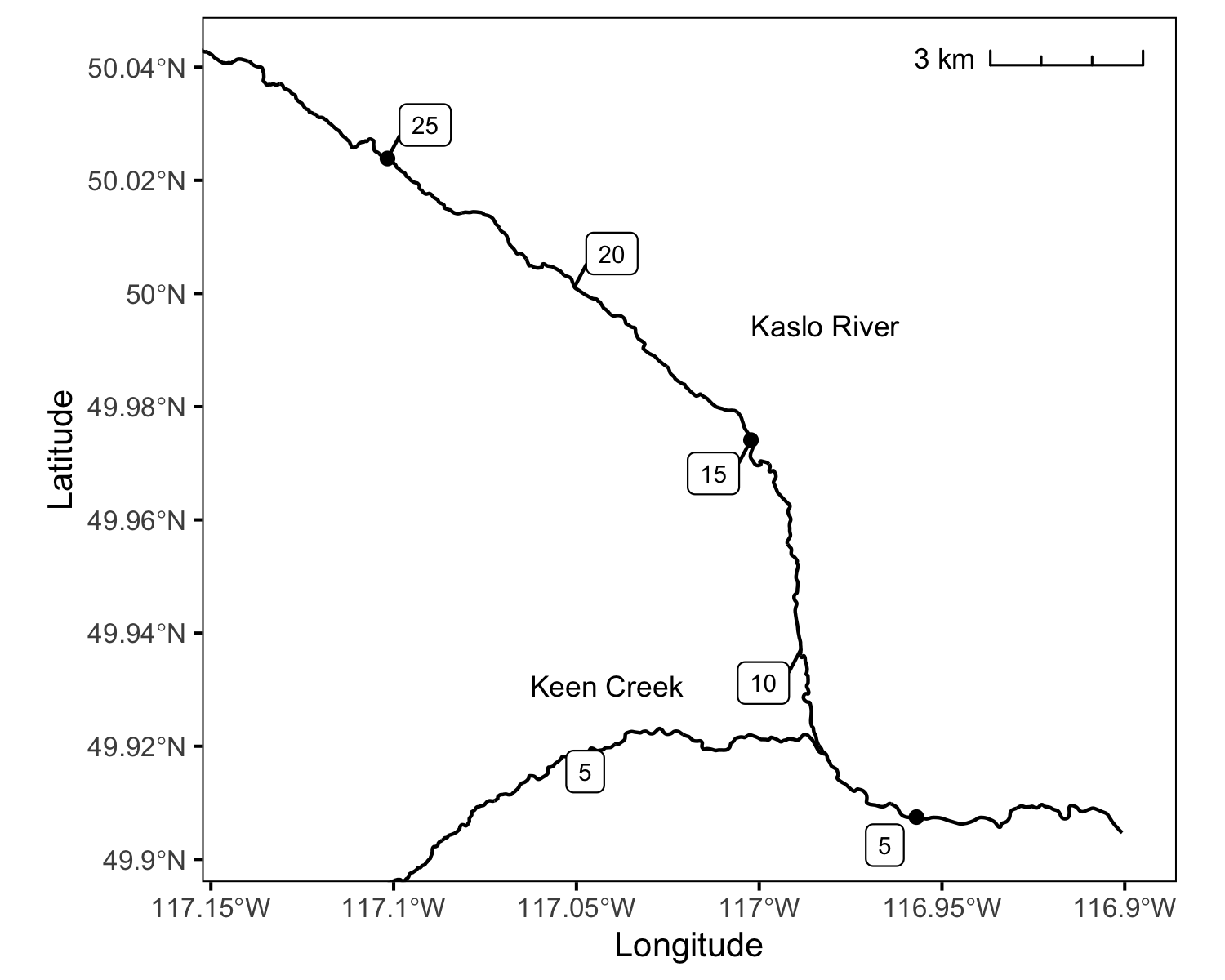Figure 1. Spatial distribution of fish-bearing channel.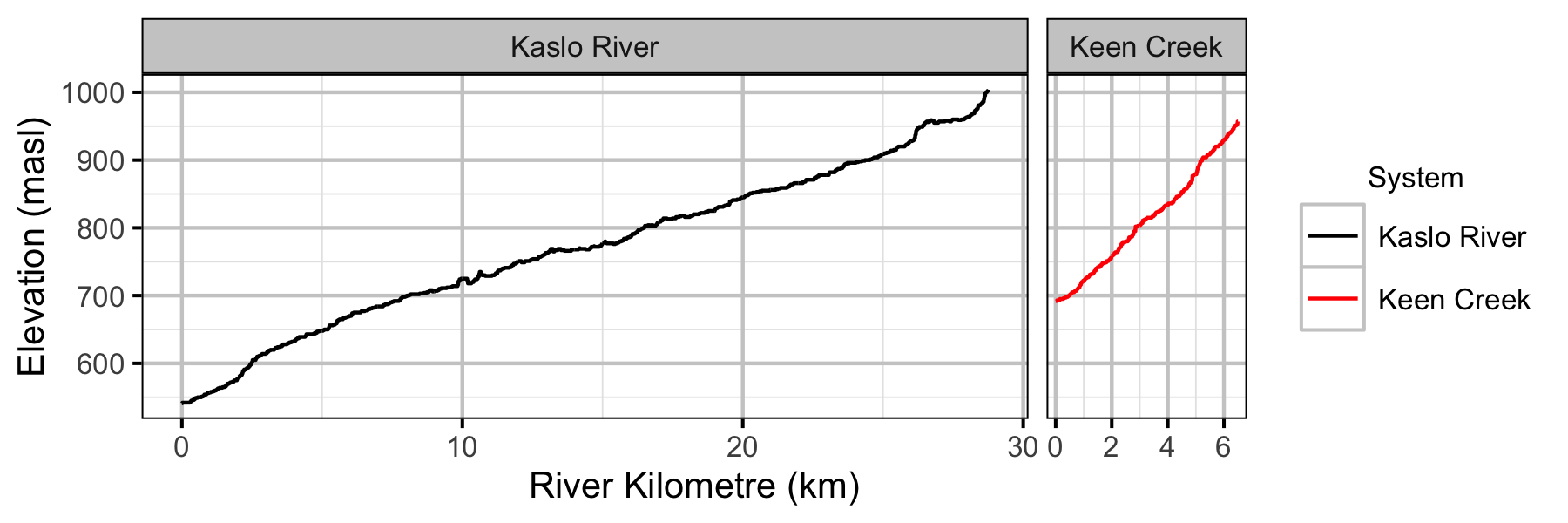Figure 2. Channel elevation by river kilometre and system.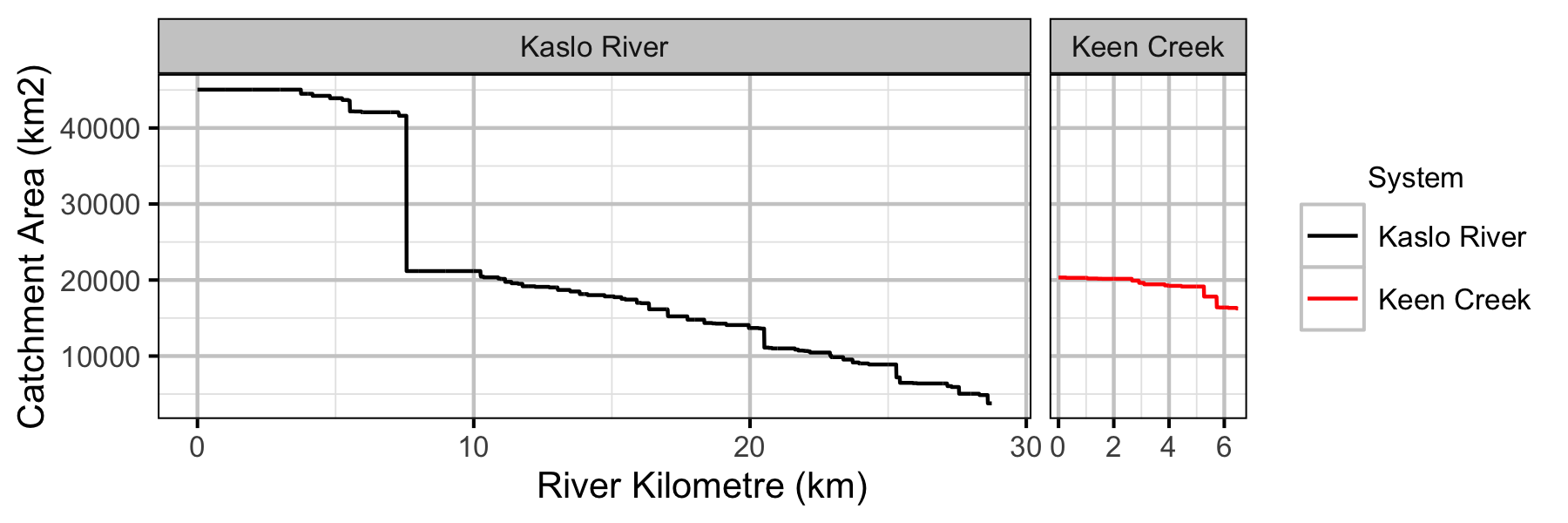Figure 3. Catchment area by river kilometre and system.

#### Sites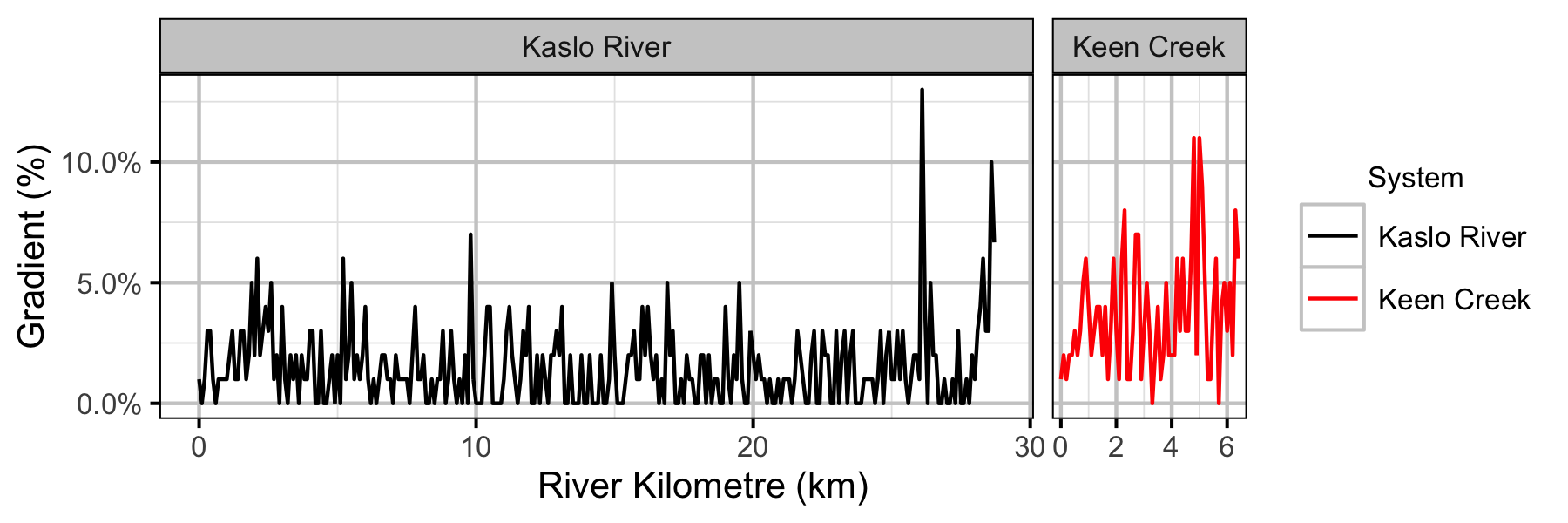Figure 4. Site gradient by river kilometre and system.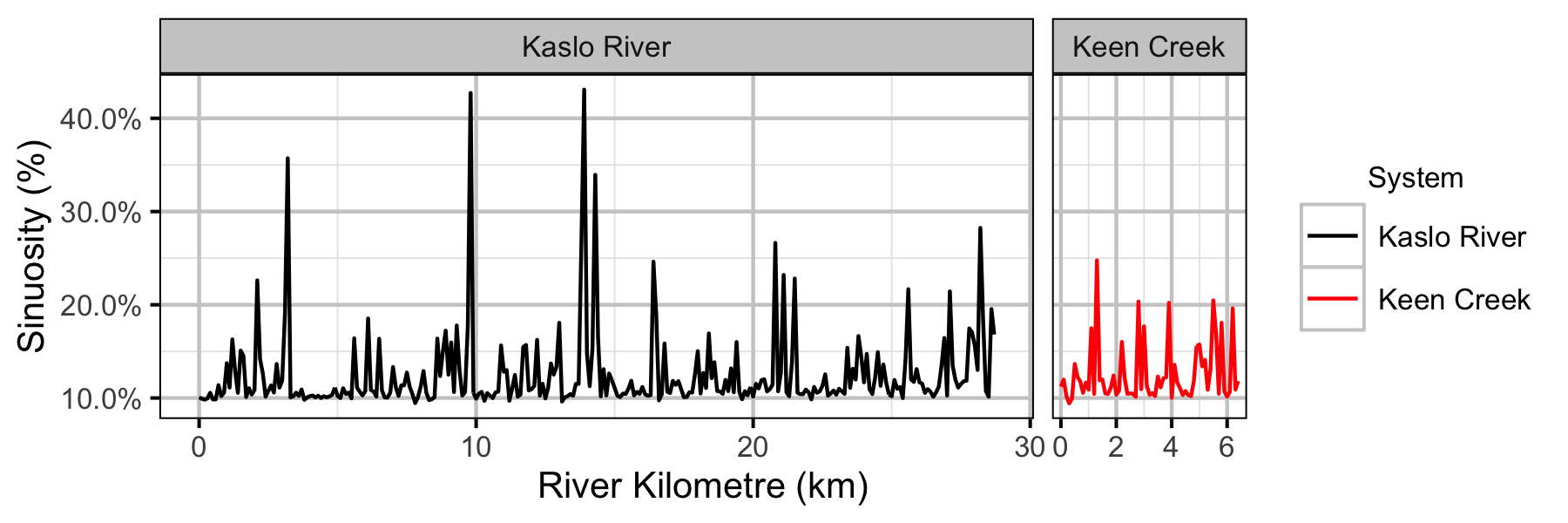Figure 5. Site sinuosity by river kilometre and system.

#### Coverage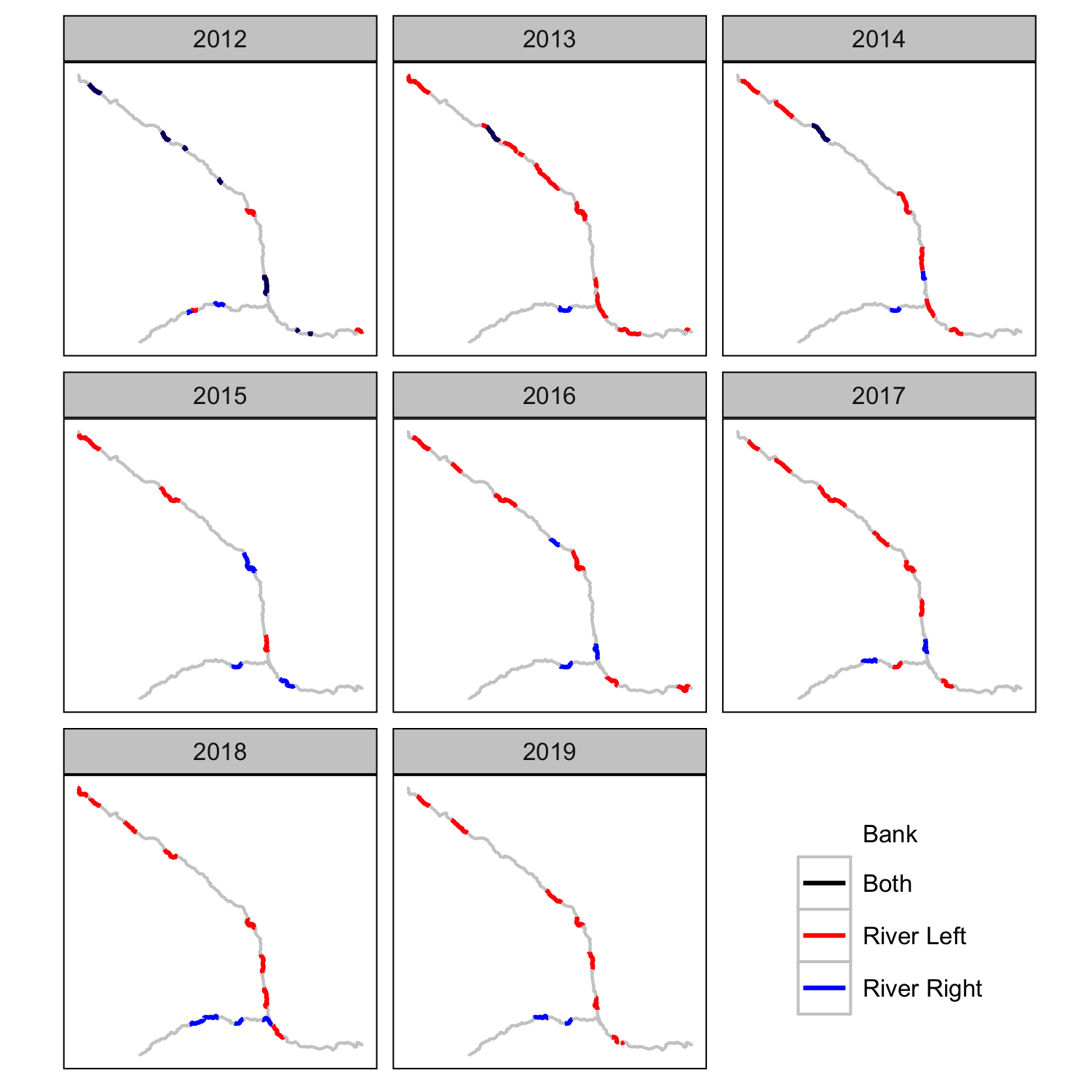Figure 6. Snorkel count coverage by year and bank.

#### Length Correction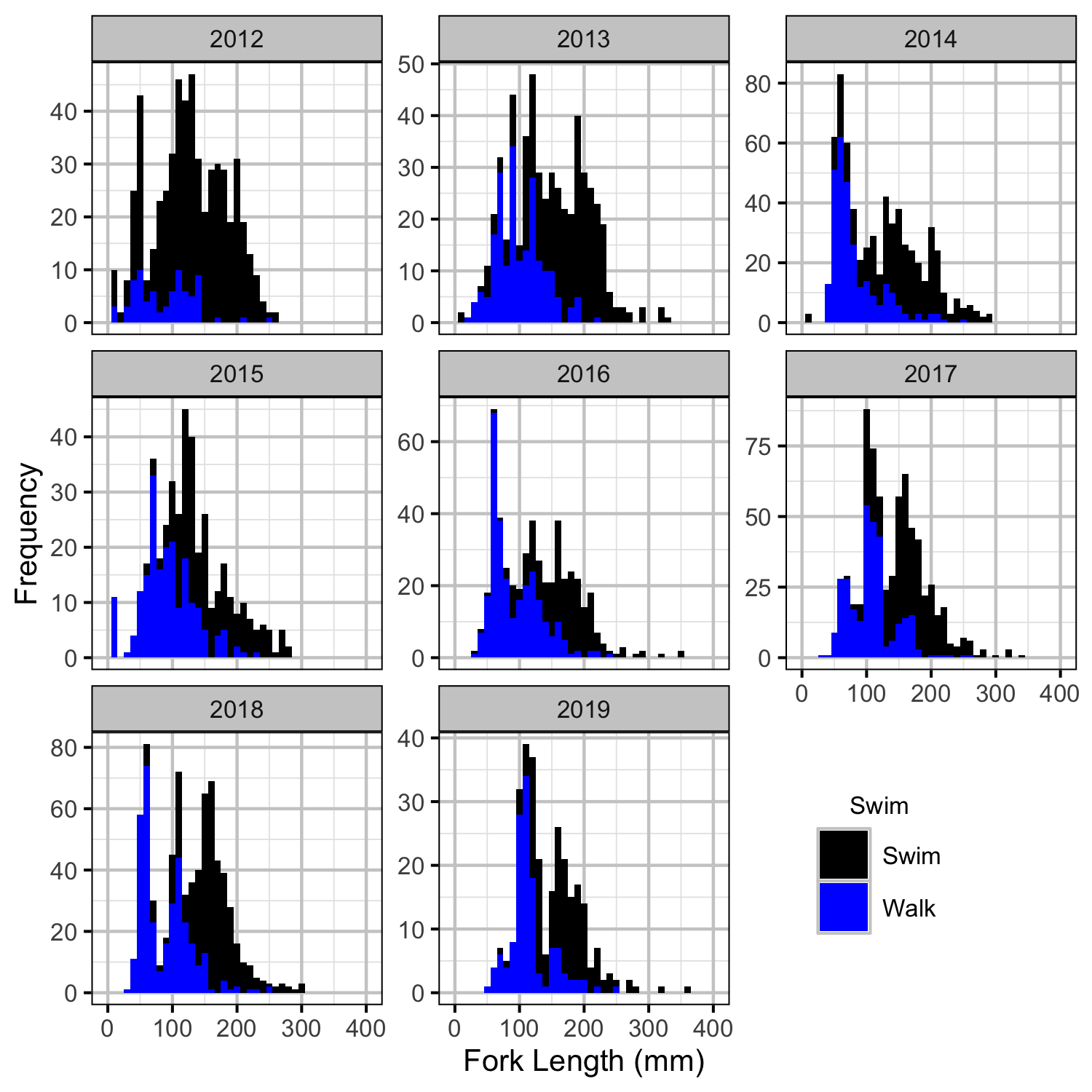Figure 7. Corrected length-frequency histogram by year and observation type.

#### Fish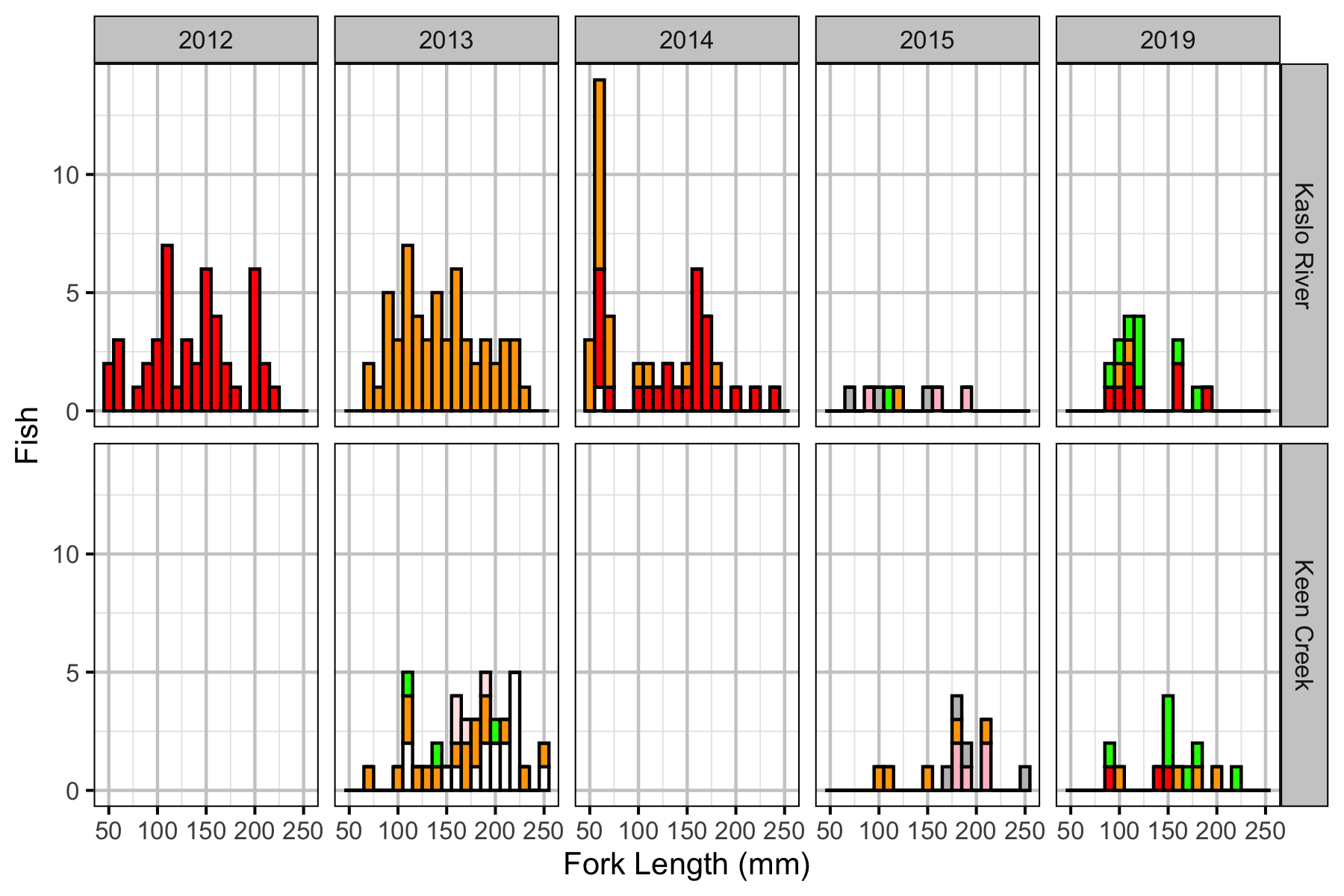Figure 8. Length-frequency plot of marked Bull Trout by year and system.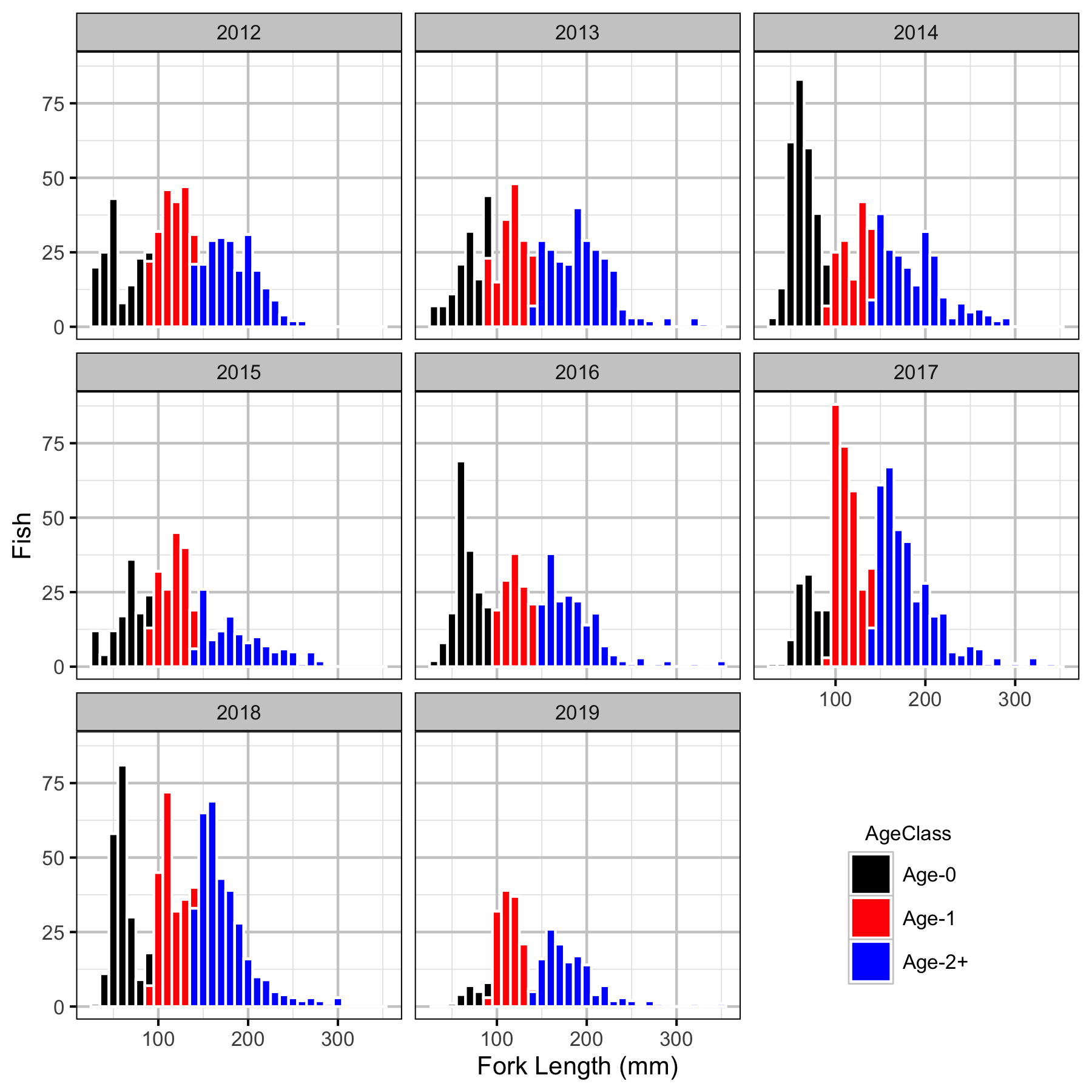Figure 9. Length-frequency plot of observed Bull Trout by year.

#### Observer Efficiency

##### Bayesian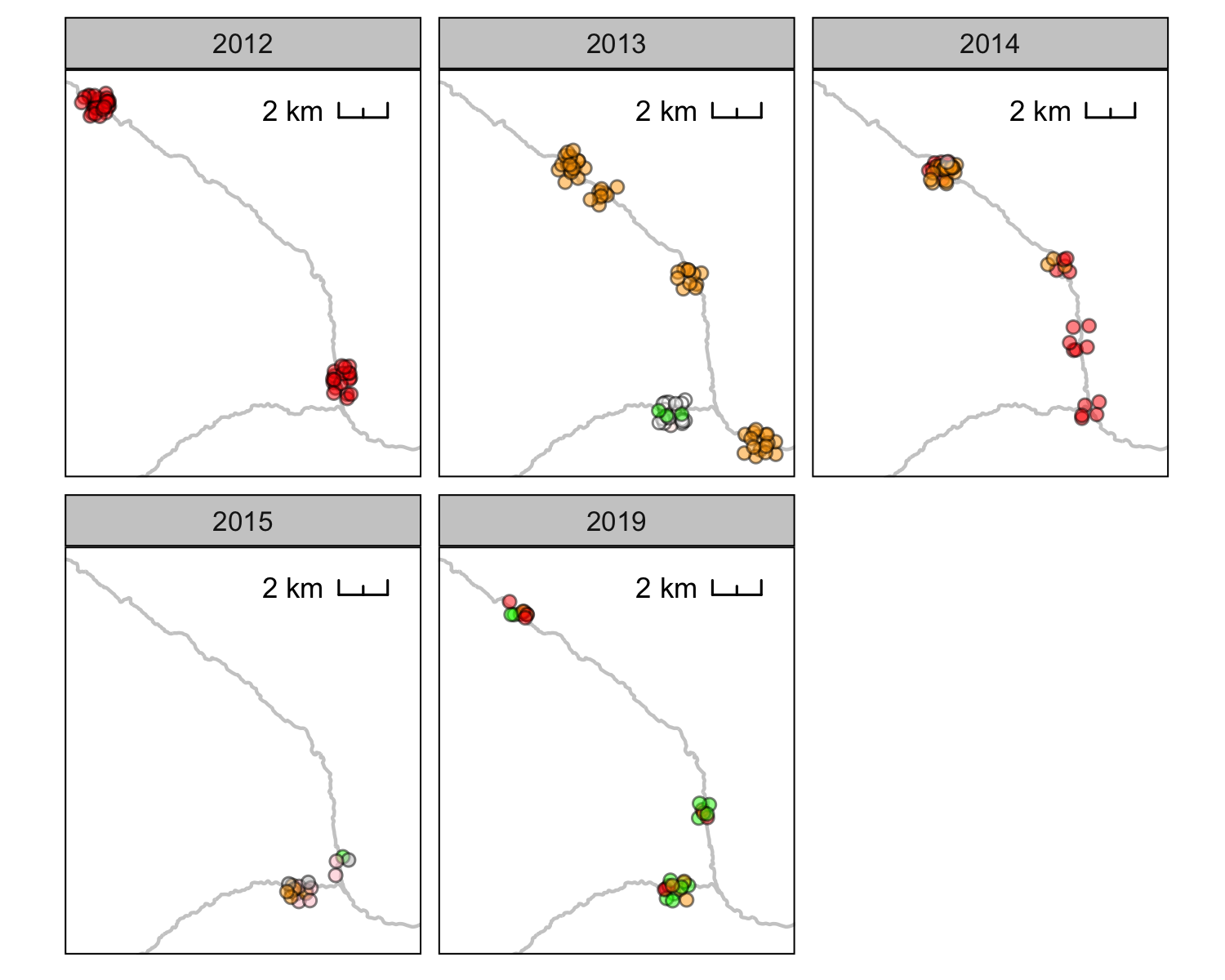Figure 10. Distribution of marked juvenile Bull Trout by year and tag color.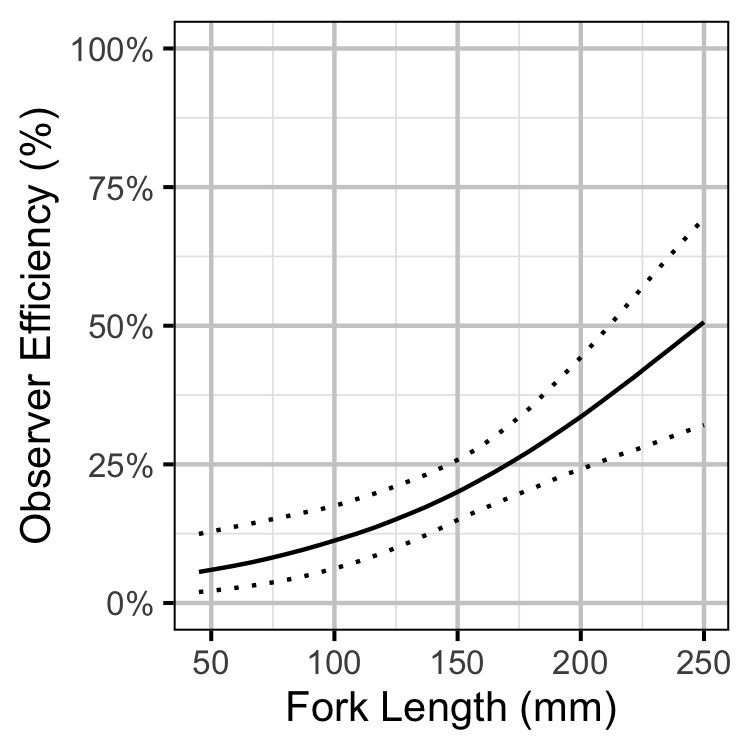Figure 11. Estimated observer efficiency by fork length and system (with 95% CIs).

#### Lineal Density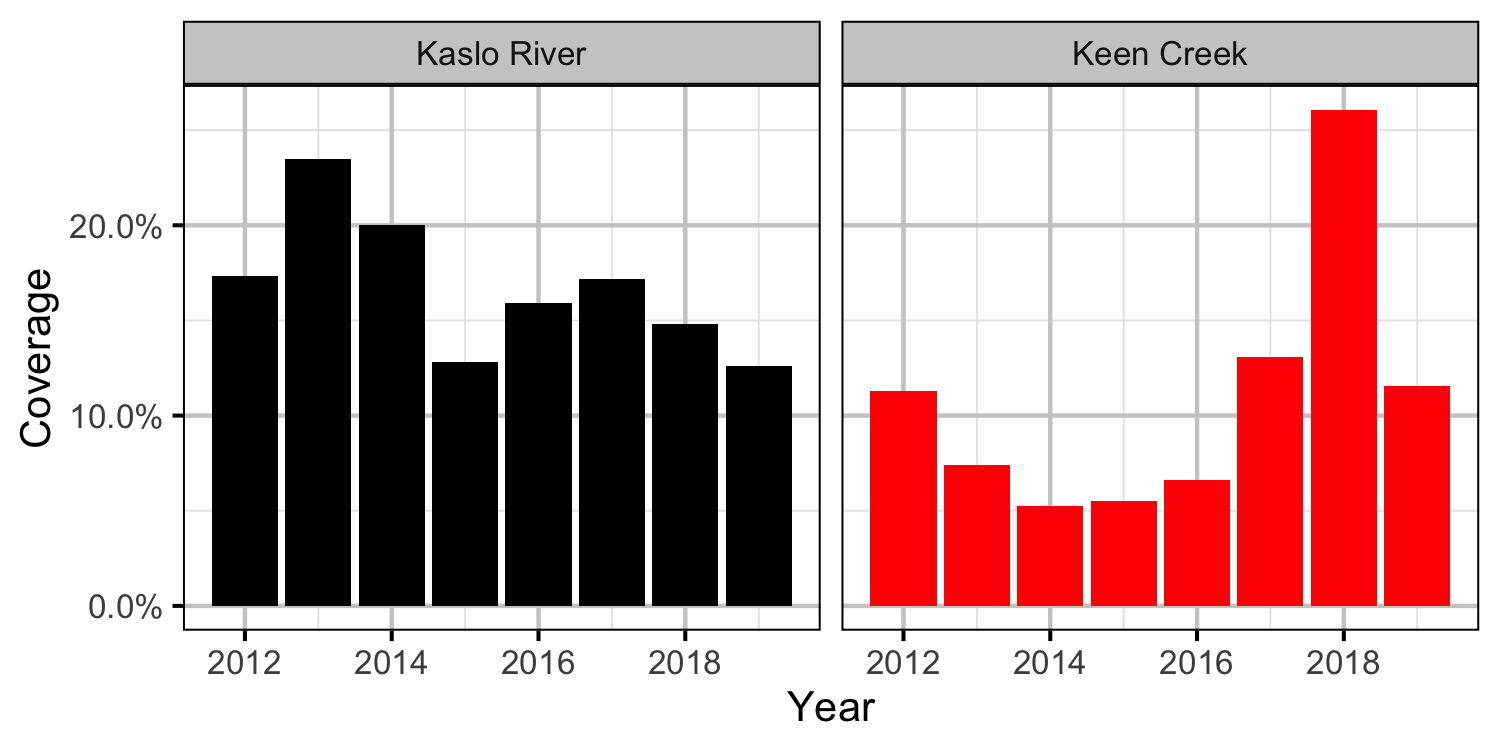Figure 12. Percent coverage by year and system.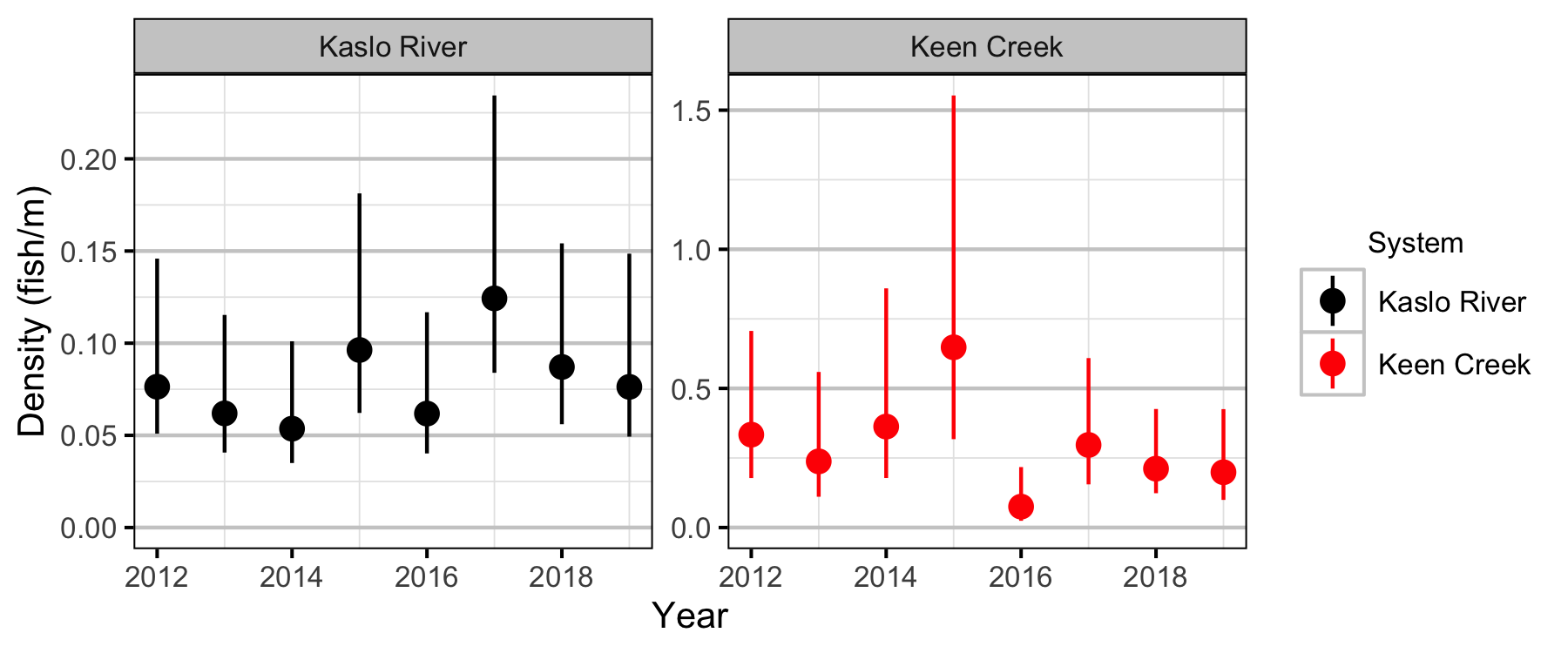Figure 13. Estimated density by year and system (with 95% CIs).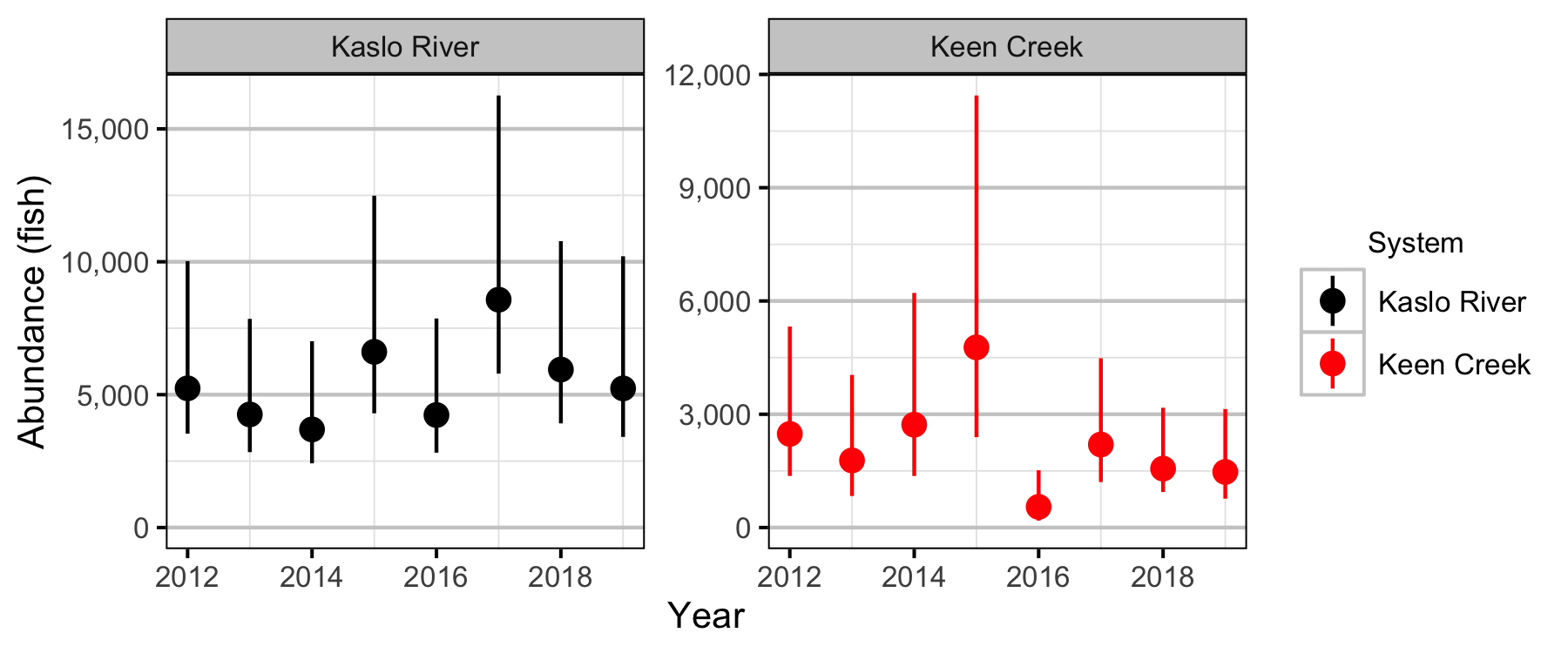Figure 14. The estimated abundance by year and system (with 95% CIs).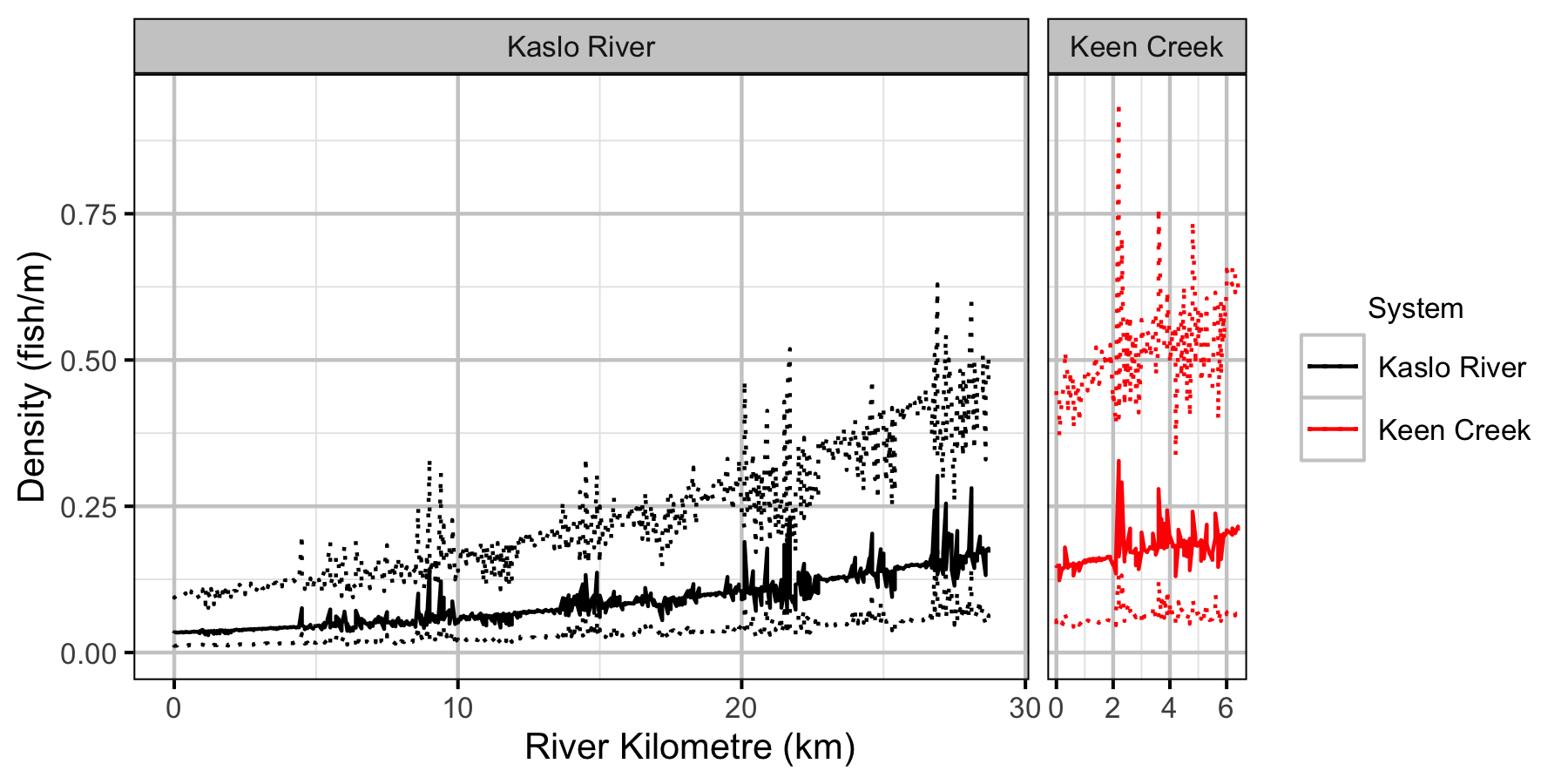Figure 15. The estimated density by site and system (with 95% CIs).

#### Stock-Recruiment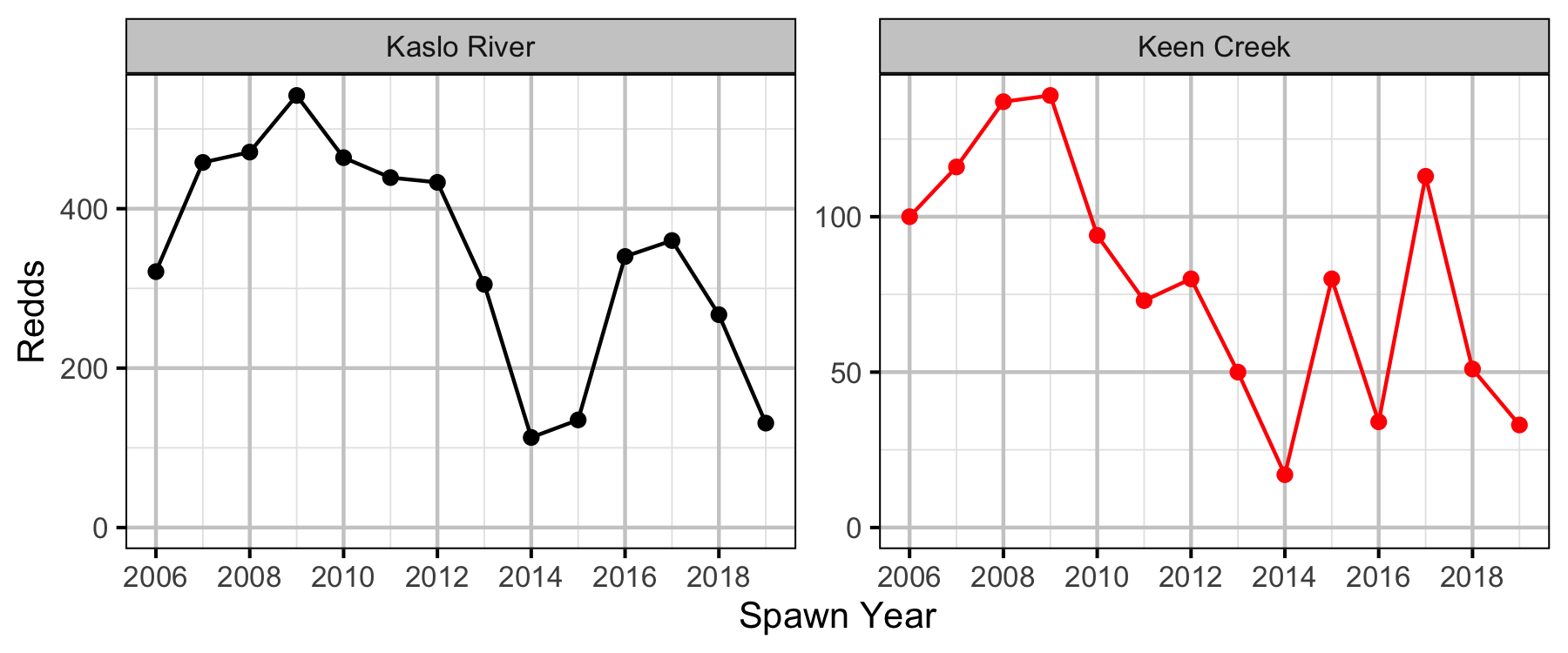Figure 16. Complete redds by system and spawn year.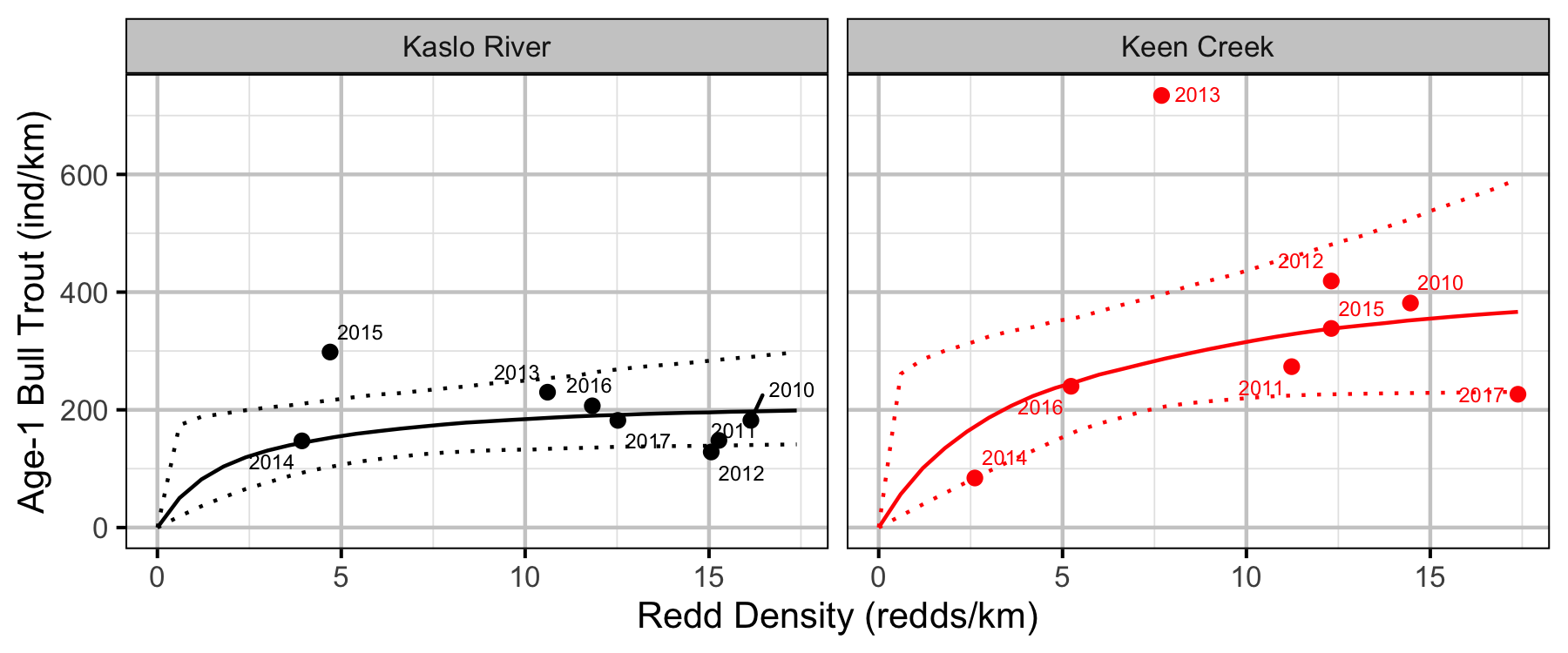Figure 17. Estimated stock-recruitment relationship (with 95% CIs). The points are labelled by spawn year.

## Conclusions

• Observer efficiency is approximately 17% for age-1 Bull Trout.
• Age-1 Bull Trout are relatively evenly distributed with respect to mesohabitat.
• Lineal densities of age-1 Bull Trout increase with river kilometre in both systems.
• The age-1 carrying capacity is estimated to be around 220 fish per km in Kaslo River and around 440 fish per km in Keen Creek.

## Acknowledgements

The organisations and individuals whose contributions have made this analysis report possible include:

• Habitat Conservation Trust Foundation
• Ministry of Forest, Lands and Natural Resource Operations
• Greg Andrusak
• Ministry of Environment
• Jen Sarchuk
• BC Fish and Wildlife
• Trina Radford
• Stephan Himmer
• Gillian Sanders
• Jeff Berdusco
• Vicky Lipinski
• Jimmy Robbins
• Jason Bowers